# A.3 Affine transforms

An affine transform is a generalization of a rigid transform, in which the rotational component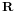is replaced by a general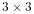matrix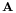. This means that an affine transform implements a generalized basis transformation combined with an offset of the origin (Figure A.7). As withfor rigid transforms, the columns ofstill describe the transformed basis vectors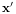,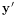, and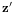, but these are generally no longer orthonormal.Figure A.7: A position vector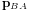and a general matrix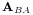describing the affine position and basis transform of frame B with respect to frame A.

Expressed in terms of homogeneous coordinates, the affine transform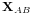takes the form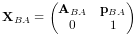(A.18)

with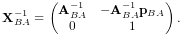(A.19)

As with rigid transforms, when an affine transform is applied to a vector instead of a point, only the matrixis applied and the translation component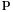is ignored.

Affine transforms are typically used to effect transformations that require stretching and shearing of a coordinate frame. By the polar decomposition theorem,can be factored into a regular rotationplus a symmetric shearing/scaling matrix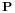: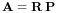(A.20)

Affine transforms can also be used to perform reflections, in whichis orthogonal (so that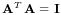) but with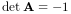.